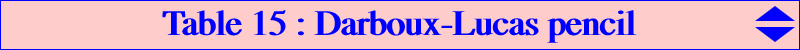The Darboux cubic K004 and the Lucas cubic K007 generate a pencil of cubics passing through A, B, C, H = X(4), L = X(20) and the four CPCC points, see Table11. This pencil contains several remarkable cubics (K) mentioned in the following table. The isogonal transform (K)* of (K) is a circum-cubic passing through X(3), X(64) and the isogonal conjugates of the four CPCC points. These cubics also belong to a same pencil.(K) remarks about (K) E (K)* remarks about (K)* (pK) K004 one of the three pKs of the pencil, a pK++ X(3) K004 one of the three pKs of the pencil, a pK++ K004 K007 one of the three pKs of the pencil X(2) K172 one of the three pKs of the pencil K002 K032 X(20) K156 K122 also member of the pencil K005 - K060 X(5) K633 also member of the pencil K005 - K073 K006 K268 the only K60 of the pencil, a stelloid X(140) CircumNormal cubic / 3, 64, 1173, 6759 K003 K313 the only circular cubic of the pencil X(30) K523 the only circular cubic of the pencil K001 K329 one of the three pKs of the pencil, a pK+ X(4) one of the three pKs of the pencil pK(X6,X3146) K522 X(631) 3, 64, 3527 pK(X6,X3522) K948 X(3628) 3, 61, 62, 64, 10282 K005 4, 20, 1371, 1372, 3830, 14226, 14241 X(3830) 3, 64, 6221, 6398 pK(X6, X15640) 4, 20, 1373, 1374, 3316, 3317, 5070 X(5070) 3, 64, 3311, 3312 pK(X6,X5056) 4, 20, 226, 1751, 3668, 6678 X(6678) 3, 64, 284, 579, 2328 K109 4, 20, 76, 83, 3674, 7819 X(7819) 3, 32, 39, 64 K020Any cubic (K) meets the Euler line at X(4), X(20) and a third point E. Let X be the anticomplement of the anticomplement of E and (pK) the member of the Euler pencil pK(X6, X). (K) belongs to the pencil generated by (pK) and the union of the line at infinity (L) with the Kiepert hyperbola (H). (K)* belongs to the pencil generated by (pK) and the union of the circumcircle (O) with the Brocard axis (B). Note that (K) is in fact the Orion cubic K(E) as in Table 11. *** If (K) is not the Darboux cubic K004, the cubics (K) and (K)* are distinct and then they generate a pencil of circum-cubics that contains : • K019 = nK0(X6, X647), the locus of foci of inscribed conics with center on the Brocard axis, • an isogonal pK whose pivot Q lies on the line passing through X(i) for i = 20, 3917, 5907, 11381, 11444, 11469, 11821, 13348, 14826, 15305. These pKs form a pencil and their two last (not always real) common points are isogonal conjugates on the previous line and obviously on K004.Generalization The notations are those of Table 11. Let P be a point, K(P) the corresponding Orion cubic, K(P)* its isogonal transform. When P is not the circumcenter O, these two cubics are distinct hence they generate a pencil of circum-cubics that contains : • one isogonal pK whose pivot is the midpoint of aaP and OP(P). • one focal isogonal nK0 whose root is the isogonal conjugate of the trilinear pole of the line OP. Its singular focus F is the isogonal conjugate of the infinite point of the line 𝚫(P). Note that F also lies on Γ(P). A neat example with P = X6 : the four cubics above are K168, K233, K102, K018 respectively.